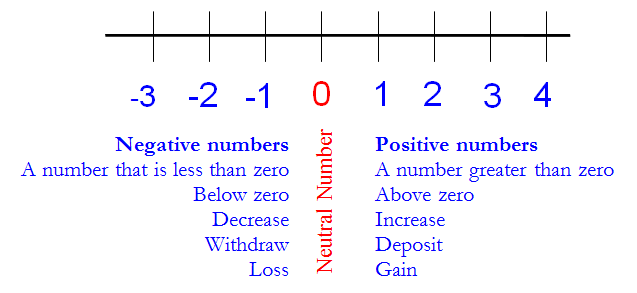# The Real Number SystemNatural Numbers:  Positive numbers from 1 and up, with no decimal or fractional part.

### 1, 2, 3, 4, 5, …

Whole Numbers: Positive numbers with no decimal or fractional part, including 0.

### 0, 1, 2, 3, 4, 5, …

Integers: All the numbers with no decimal or fractional part (positive or negative).

### …-5, -4, -3, -2, -1,0, 1, 2, 3, 4, 5, …

Rational numbers:  any number that can be expressed as the quotient or fraction p/q of two integers, (i.e. as a ratio). Since q may be equal to 1, every integer is a rational number.  q is a natural number (non-zero) denominator.

When we divide numerator between denominator of a fractional number, we can have two cases: A decimal with a finite number of digits. (terminating decimal), or a decimal that repeats over and over again indefinitely (repeating decimal).

1/2 can be represented as 0.5   (terminating decimal)

1/3 can be represented as 0.333333……. (repeating decimal)

A bar notation indicates that a number repeats forever. (repeating decimal)Irrational numbers: any number that cannot be exactly expressed as a ratio of two integers.

Real Numbers: All the numbers that can be represented on a number line.

##The Real Number System

Natural Numbers: { 1, 2, 3, 4, 5, …} or Counting Numbers

Whole Numbers: {0, 1, 2, 3, 4, 5,…}

Integers: {… -4, -3, -2, -1, 0, 1, 2, 3, 4, }Includes positive numbers and negative numbers. Also the neutral number zeroRationals_W        Rationals Writing

Integers_W      Integers WritingReal Numbers Vocabulary

Number Line

A number line is a way we can graphically represent real numbers.

Each point of the number line corresponds to a real number and vice versa.  There is an arbitrary point that we mark as zero and we call it origin.

The positive numbers are represented to the right of the origin. The negative numbers are represented to the left of the origin.

Small marks are drawn at regular intervals; and below each mark, a progressive number is written starting from 0 (the origin).

A number line is a basic tool for teaching number concepts, such as comparing, ordering, addition, subtraction, multiplication, division, rounding, and so on.Number_Line_(Help)